# Chapter 11 Inference for Distributions of Categorical Data

• Slides: 29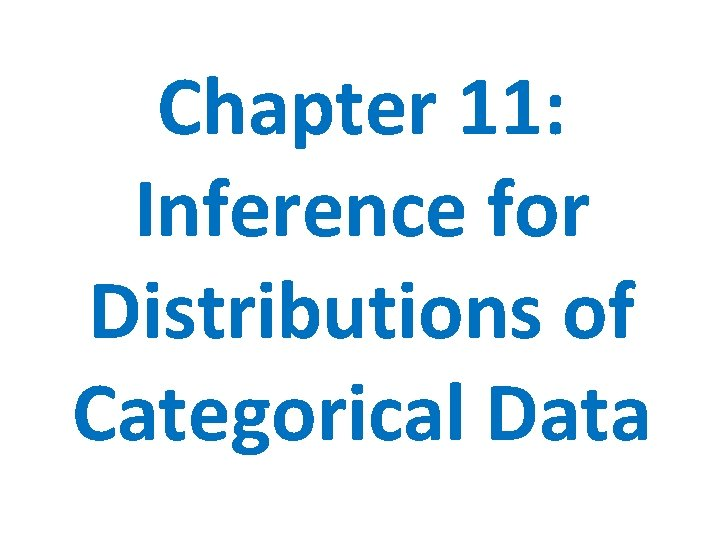Chapter 11: Inference for Distributions of Categorical Data11. 1 Chi-Square Goodness-of-Fit TestsSection 11. 1 Chi-Square Goodness-of-Fit Tests ü COMPUTE expected counts, conditional distributions, and contributions to the chi-square statistic ü CHECK the Random, Large sample size, and Independent conditions before performing a chi-square test ü PERFORM a chi-square goodness-of-fit test to determine whether sample data are consistent with a specified distribution of a categorical variable ü EXAMINE individual components of the chi-square statistic as part of a follow-up analysisIntroduction Input/Desired Result Test Required One Categorical Variable Chi-Square Goodness of Fit (GOF) Two Categorical Variables Chi-Square Test for Homogeneity Study Relationship between Chi-Square Test for Two Categorical Variables Association/Independence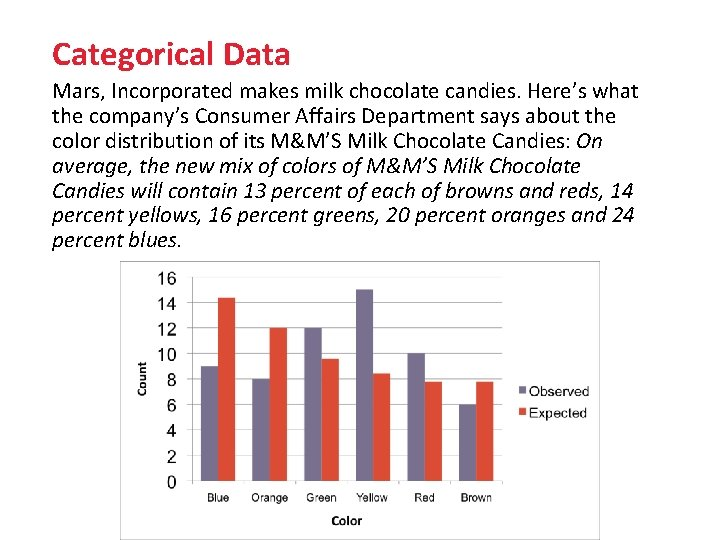Categorical Data Mars, Incorporated makes milk chocolate candies. Here’s what the company’s Consumer Affairs Department says about the color distribution of its M&M’S Milk Chocolate Candies: On average, the new mix of colors of M&M’S Milk Chocolate Candies will contain 13 percent of each of browns and reds, 14 percent yellows, 16 percent greens, 20 percent oranges and 24 percent blues.WHY? Chi-Square Goodness-of-Fit Tests The one-way table below summarizes the data from a sample bag of M&M’S Milk Chocolate Candies. Color Blue Orange Green Yellow Red Brown Total Count 9 8 12 15 10 6 60 Since the company claims that 24% of all M&M’S Milk Chocolate Candies are blue, we might believe that something fishy is going on. We could use the one-sample z test for a proportion from Chapter 9 to test the hypotheses H 0: p = 0. 24 Ha: p ≠ 0. 24 However, performing a one-sample z test for each proportion would be pretty inefficient and would lead to the problem of multiple comparisons.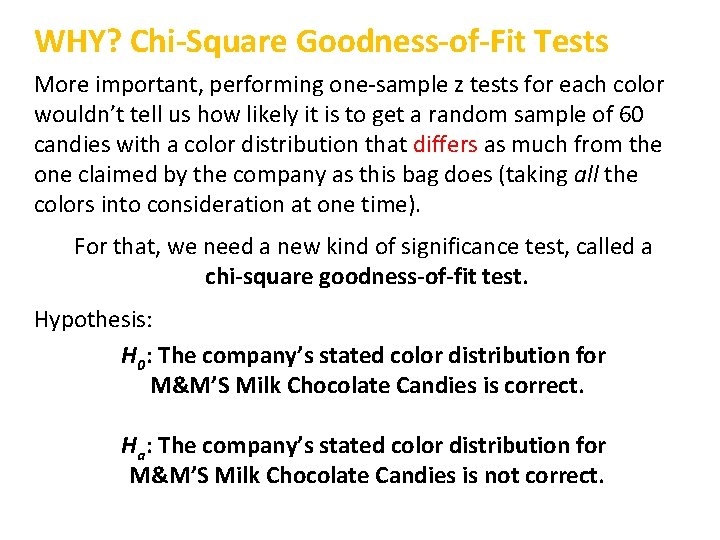WHY? Chi-Square Goodness-of-Fit Tests More important, performing one-sample z tests for each color wouldn’t tell us how likely it is to get a random sample of 60 candies with a color distribution that differs as much from the one claimed by the company as this bag does (taking all the colors into consideration at one time). For that, we need a new kind of significance test, called a chi-square goodness-of-fit test. Hypothesis: H 0: The company’s stated color distribution for M&M’S Milk Chocolate Candies is correct. Ha: The company’s stated color distribution for M&M’S Milk Chocolate Candies is not correct.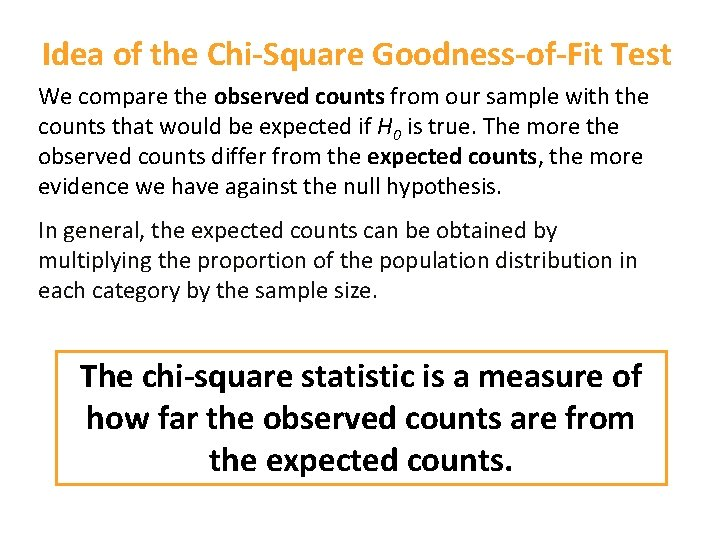Idea of the Chi-Square Goodness-of-Fit Test We compare the observed counts from our sample with the counts that would be expected if H 0 is true. The more the observed counts differ from the expected counts, the more evidence we have against the null hypothesis. In general, the expected counts can be obtained by multiplying the proportion of the population distribution in each category by the sample size. The chi-square statistic is a measure of how far the observed counts are from the expected counts.The Chi-Square Statistic To see if the data give convincing evidence against the null hypothesis, we compare the observed counts from our sample with the expected counts assuming H 0 is true. If the observed counts are far from the expected counts, that’s the evidence we were seeking. Big Chi-Square values means the data is far from what we are expecting, giving us evidence against the null (reject). The chi-square statistic is a measure of how far the observed counts are from the expected counts. The formula for the statistic isCalculating Expected Values For random samples of 60 candies, the average number of blue M&M’s should be (0. 24)(60) = 14. 40. This is our expected count of blue M&M’s. Using this same method, we can find the expected counts for the other color categories: Proportions According to Mars Orange: (0. 20)(60) = 12. 00 Green: (0. 16)(60) = 9. 60 Yellow: (0. 14)(60) = 8. 40 Red: (0. 13)(60) = 7. 80 Brown: (0. 13)(60) = 7. 80Calculating Expected Values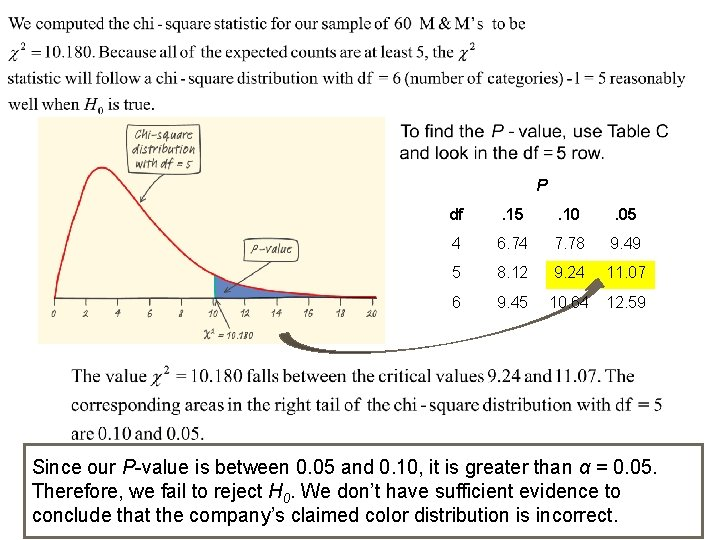P df . 15 . 10 . 05 4 6. 74 7. 78 9. 49 5 8. 12 9. 24 11. 07 6 9. 45 10. 64 12. 59 Since our P-value is between 0. 05 and 0. 10, it is greater than α = 0. 05. Therefore, we fail to reject H 0. We don’t have sufficient evidence to conclude that the company’s claimed color distribution is incorrect.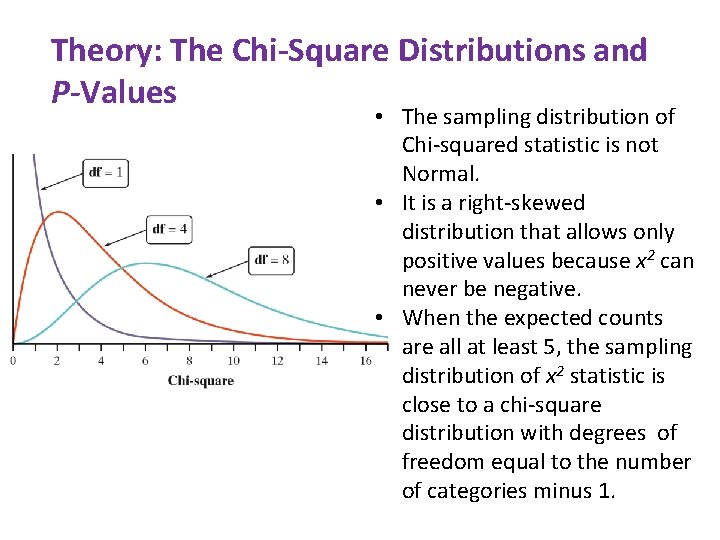Theory: The Chi-Square Distributions and P-Values • The sampling distribution of Chi-squared statistic is not Normal. • It is a right-skewed distribution that allows only positive values because x 2 can never be negative. • When the expected counts are all at least 5, the sampling distribution of x 2 statistic is close to a chi-square distribution with degrees of freedom equal to the number of categories minus 1.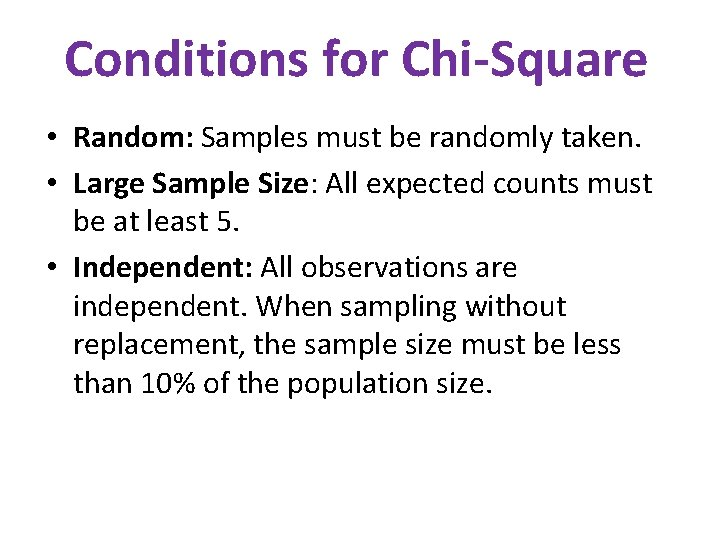Conditions for Chi-Square • Random: Samples must be randomly taken. • Large Sample Size: All expected counts must be at least 5. • Independent: All observations are independent. When sampling without replacement, the sample size must be less than 10% of the population size.Using your TI-Nspire: 1. Enter the observed and expected data in the spreadsheet. 2. Label the 1 st column: mmobs and 2 nd column: mmexp.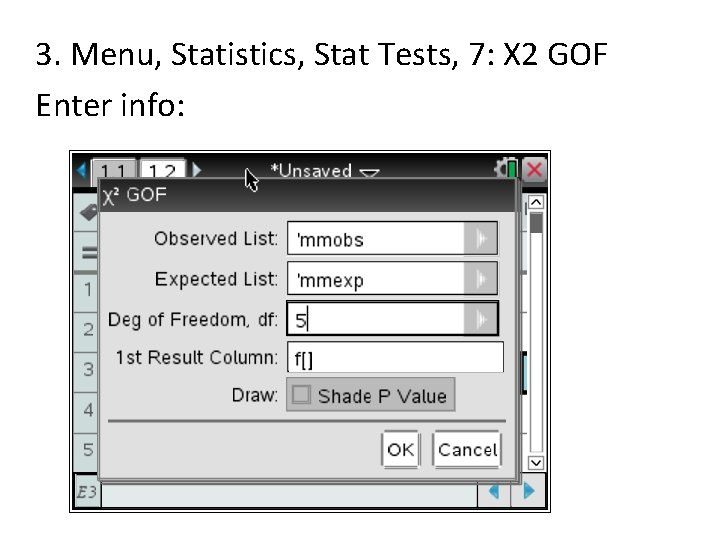3. Menu, Statistics, Stat Tests, 7: X 2 GOF Enter info: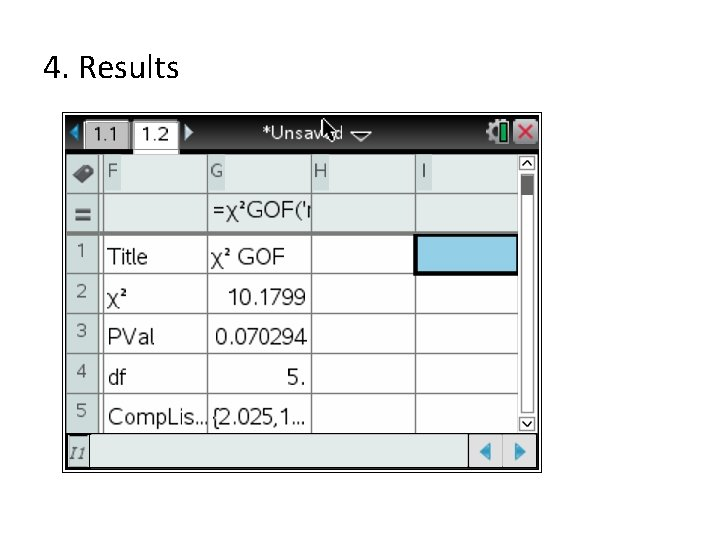4. Results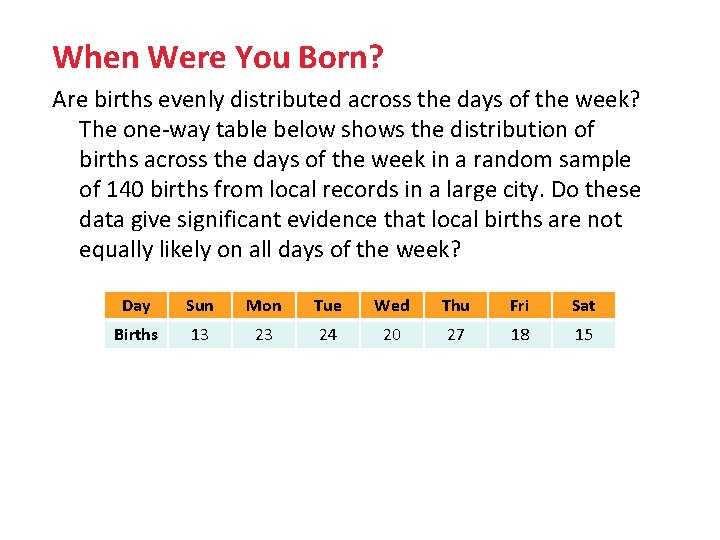When Were You Born? Are births evenly distributed across the days of the week? The one-way table below shows the distribution of births across the days of the week in a random sample of 140 births from local records in a large city. Do these data give significant evidence that local births are not equally likely on all days of the week? Day Sun Mon Tue Wed Thu Fri Sat Births 13 23 24 20 27 18 15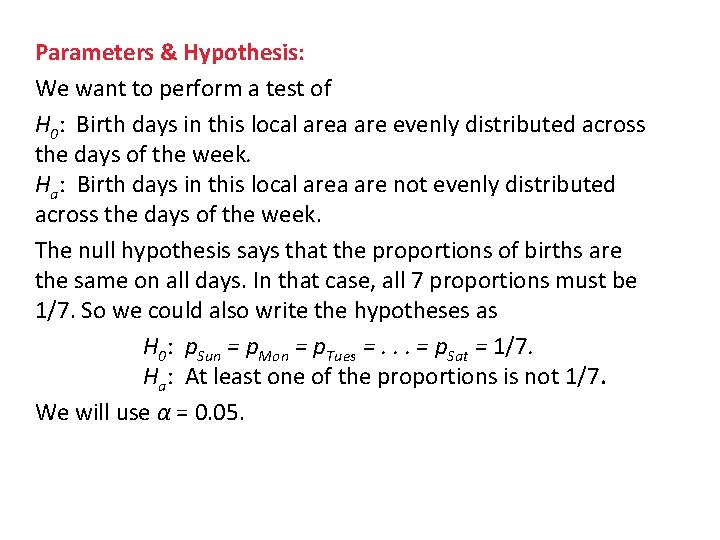Parameters & Hypothesis: We want to perform a test of H 0: Birth days in this local area are evenly distributed across the days of the week. Ha: Birth days in this local area are not evenly distributed across the days of the week. The null hypothesis says that the proportions of births are the same on all days. In that case, all 7 proportions must be 1/7. So we could also write the hypotheses as H 0: p. Sun = p. Mon = p. Tues =. . . = p. Sat = 1/7. Ha: At least one of the proportions is not 1/7. We will use α = 0. 05.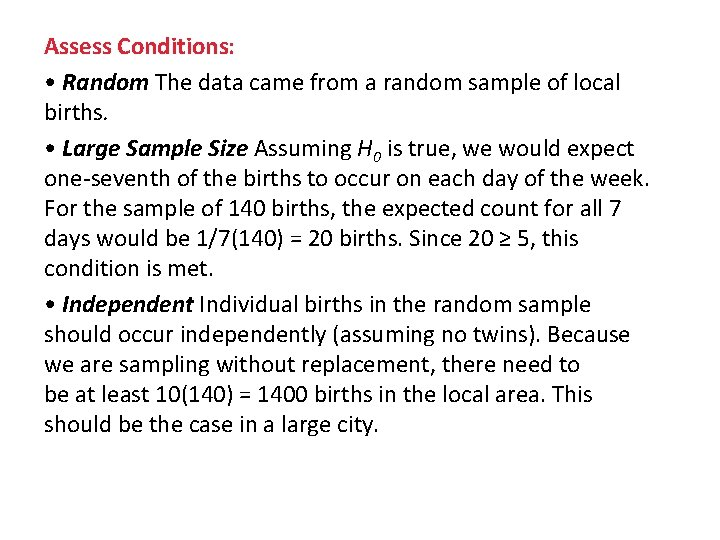Assess Conditions: • Random The data came from a random sample of local births. • Large Sample Size Assuming H 0 is true, we would expect one-seventh of the births to occur on each day of the week. For the sample of 140 births, the expected count for all 7 days would be 1/7(140) = 20 births. Since 20 ≥ 5, this condition is met. • Independent Individual births in the random sample should occur independently (assuming no twins). Because we are sampling without replacement, there need to be at least 10(140) = 1400 births in the local area. This should be the case in a large city.Name Test & (Calculate) Test Statistic Name Test: Chi-Square goodness-of-fit test.Obtain P Value P-value = 0. 269.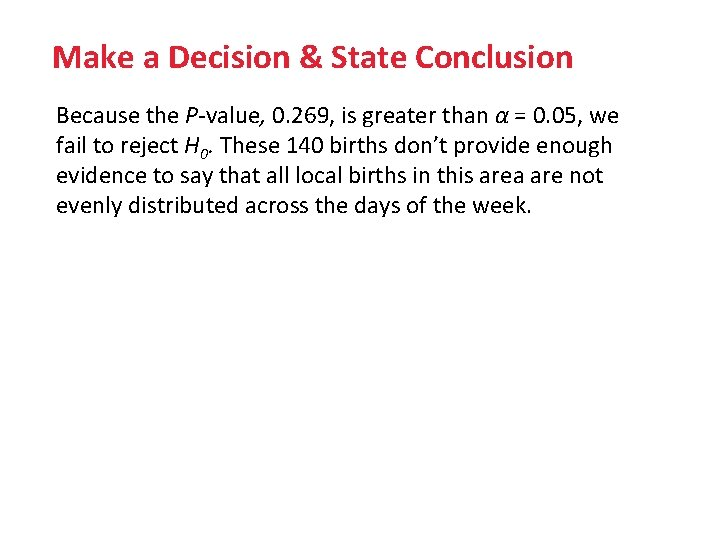Make a Decision & State Conclusion Because the P-value, 0. 269, is greater than α = 0. 05, we fail to reject H 0. These 140 births don’t provide enough evidence to say that all local births in this area are not evenly distributed across the days of the week.Inherited Traits Biologists wish to cross pairs of tobacco plants having genetic makeup Gg, indicating that each plant has one dominant gene (G) and one recessive gene (g) for color. In other words, the biologists predict that 25% of the offspring will be green, 50% will be yellow-green and 25% will be albino. Do these data differ significantly from what the biologists have predicted? Carry out an appropriate test at the α = 0. 05 level to help answer this question.Parameters & Hypothesis: H 0: The biologists’ predicted color distribution for tobacco plant offspring is correct. That is, pgreen = 0. 25, pyellow-green = 0. 5, palbino = 0. 25 Ha: The biologists’ predicted color distribution isn’t correct. That is, at least one of the stated proportions is incorrect. We will use α = 0. 05.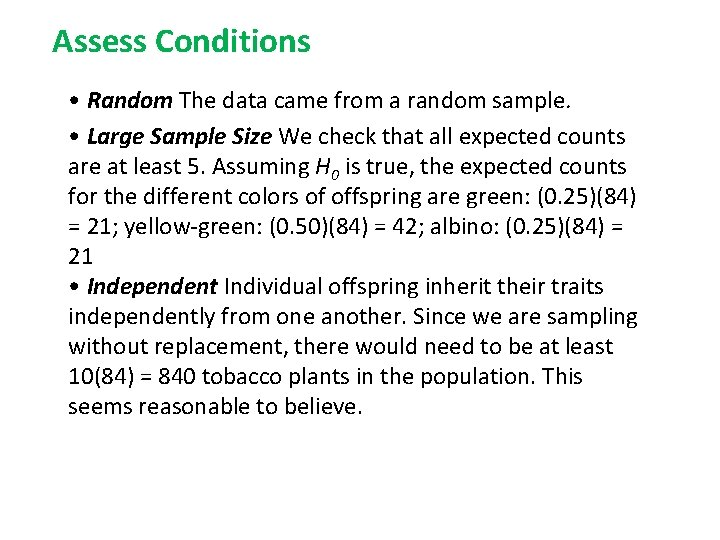Assess Conditions • Random The data came from a random sample. • Large Sample Size We check that all expected counts are at least 5. Assuming H 0 is true, the expected counts for the different colors of offspring are green: (0. 25)(84) = 21; yellow-green: (0. 50)(84) = 42; albino: (0. 25)(84) = 21 • Independent Individual offspring inherit their traits independently from one another. Since we are sampling without replacement, there would need to be at least 10(84) = 840 tobacco plants in the population. This seems reasonable to believe.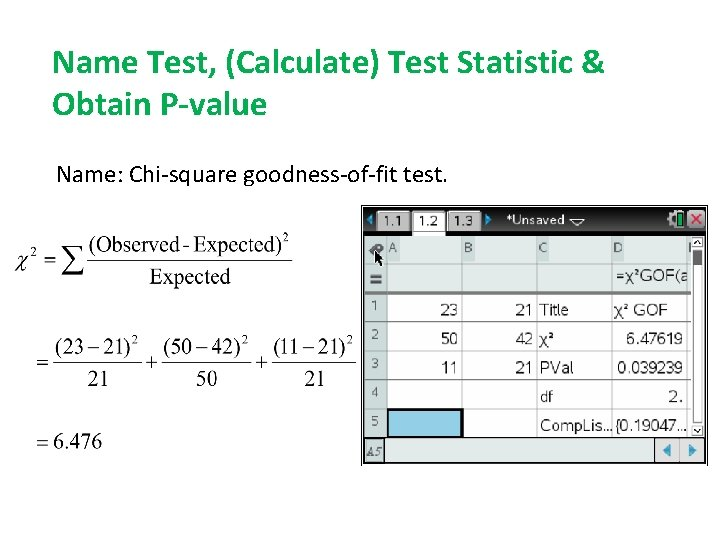Name Test, (Calculate) Test Statistic & Obtain P-value Name: Chi-square goodness-of-fit test.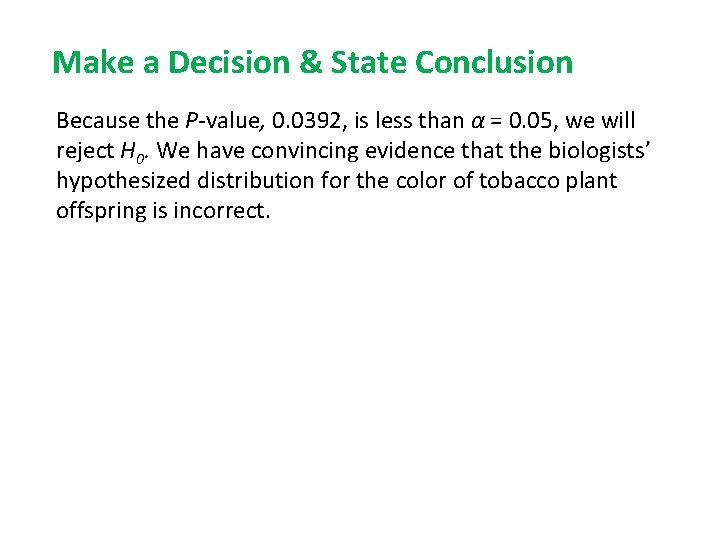Make a Decision & State Conclusion Because the P-value, 0. 0392, is less than α = 0. 05, we will reject H 0. We have convincing evidence that the biologists’ hypothesized distribution for the color of tobacco plant offspring is incorrect.WARNING!!!! In order to perform a Chi. Square Test, the data must be counts!!!!